Latest Banking jobs   »   IBPS reasoning

# Reasoning Quiz For IBPS PO Prelims 2023-05th September

Directions (1-5): Read the given series of numbers and alphabets carefully and answer the questions based on it.

W U 5 F 6 J K 9 A 3 C E 7 8 Q O 1 2 Z 4 P D A

Q1. In the given series, how many vowels are to the right of 8?
(a) One
(b) Two
(c) Three
(d) Four
(e) None of these

Q2. How many odd numbers are immediately preceded and immediately succeeded by an alphabet in the given series?
(a) One
(b) Two
(c) Three
(d) Four
(e) None of these

Q3. What is the difference between the highest and the lowest number in the given series?
(a) 5
(b) 6
(c) 7
(d) 8
(e) None of these

Q4. Which element is 10th to the right of 7th element from the left end of the given series?
(a) O
(b) 1
(c) Q
(d) 2
(e) None of these

Q5. What is the sum of 5th number from the right end and 4th number from the left end?
(a) 15
(b) 10
(c) 14
(d) 17
(e) None of these

Directions (6-10): In each of the questions below, some statements are given followed by some Conclusions. You have to take the given statements to be true even, if they seem to be at variance from commonly known facts. Read all the conclusions and then decide which of the given conclusions logically follows from the given statements disregarding commonly known facts.
(a) If only conclusion I follows
(b) If only conclusion II follows
(c) If either conclusion I or II follows
(d) If neither conclusion I nor II follows
(e) If both conclusions I and II follow

Q6. Statements:
Some Icon are Image
All Image are File
Some Folder are Icon
Conclusions:
I: Some Folder are not File
II: All Folder are File

Q7. Statements:
Some Monday are Off
Some Off are Sunday
Some Sunday are Fun
Conclusions:
I: Some Fun are not Monday
II: All Monday are Fun

Q8. Statements:
All Movie are Film
Some movie are Documentary
All Documentary are Best
Conclusions:
I. Some film are best
II. Some Documentary are Film.

Q9. Statements:
Some paper is Eraser
All Eraser is pen
All Pen are Pencil
Conclusions:
I. Some Paper are not Pencil
II. Some Paper are Pen is a possibility.

Q10. Statements:
All Style are Unique
No Music is Boring
All Boring are Unique
Conclusions:
I. Some style are not Music.
II. Some Unique are not Music

Solutions

Solutions (1-5):
S1. Ans. (b)
Sol. Given series: W U 5 F 6 J K 9 A 3 C E 7 8 Q O 1 2 Z 4 P D A
Hence, there are two vowels (O, A) are to the right of 8.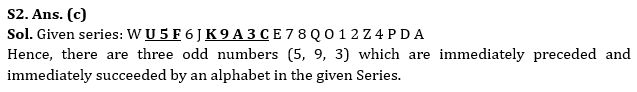S3. Ans. (d)
Sol. Given series: W U 5 F 6 J K 9 A 3 C E 7 8 Q O 1 2 Z 4 P D A
Highest number = 9, Lowest number = 1
So, required difference = 9 – 1 = 8

S4. Ans. (b)
Sol. Given series – W U 5 F 6 J K 9 A 3 C E 7 8 Q O 1 2 Z 4 P D A
7th element from the left end = K. So, 10th to the right of K = 1
Hence, 1 is the correct answer.

S5. Ans. (b)
Sol. Given series – W U 5 F 6 J K 9 A 3 C E 7 8 Q O 1 2 Z 4 P D A
5th number from the right end = 7, 4th number from the left end = 3
So, required sum = 7 + 3 = 10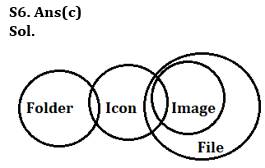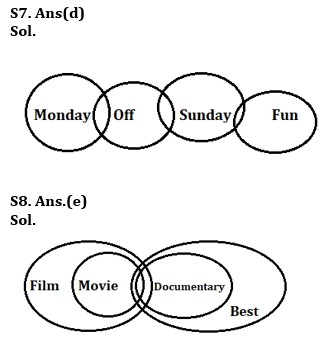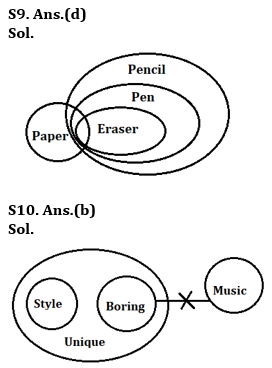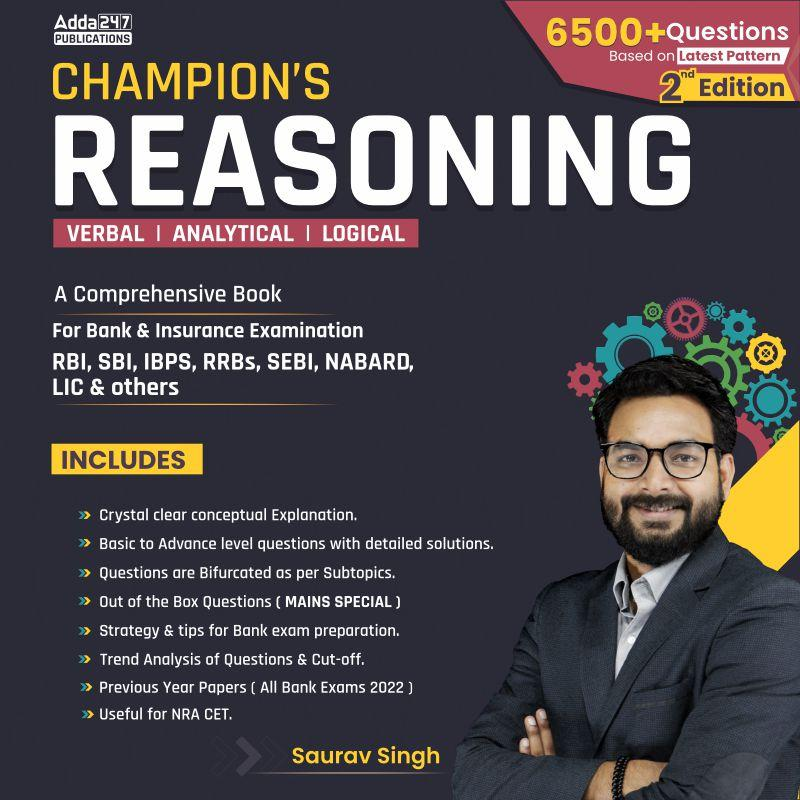## FAQs

### When will the IBPS PO prelims 2023 be conducted?

IBPS PO Prelims will be conducted on 23, 30 September, and 1 October 2023.

#### Congratulations!Union Budget 2023-24: Free PDF